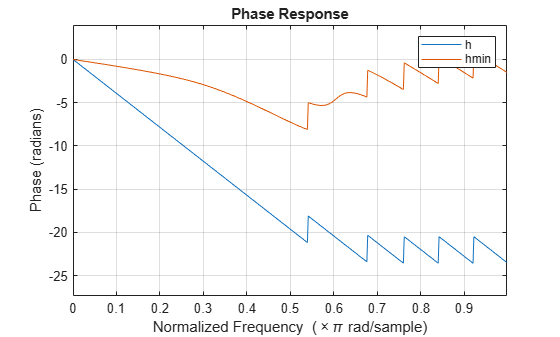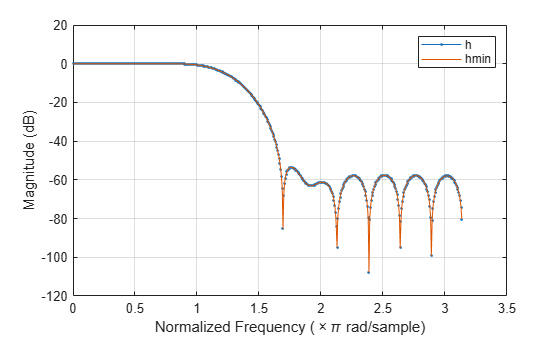Documentation

### This is machine translation

Mouseover text to see original. Click the button below to return to the English version of the page.

Note: This page has been translated by MathWorks. Click here to see
To view all translated materials including this page, select Country from the country navigator on the bottom of this page.

# polystab

Stabilize polynomial

## Syntax

```b = polystab(a) ```

## Description

`polystab` stabilizes a polynomial with respect to the unit circle; it reflects roots with magnitudes greater than 1 inside the unit circle.

`b = polystab(a)` returns a row vector `b` containing the stabilized polynomial. `a` is a vector of polynomial coefficients, normally in the z-domain:

`$A\left(z\right)=a\left(1\right)+a\left(2\right){z}^{-1}+\dots +a\left(m+1\right){z}^{-m}.$`

## Examples

collapse all

Use the window method to design a 25th-oder FIR filter with normalized cutoff frequency $0.4\pi$ rad/sample. Verify that it has linear phase but not minimum phase.

```h = fir1(25,0.4); h_linphase = islinphase(h)```
```h_linphase = logical 1 ```
`h_minphase = isminphase(h)`
```h_minphase = logical 0 ```

Use `polystab` to convert the linear-phase filter into a minimum-phase filter. Plot the phase responses of the filters.

```hmin = polystab(h)*norm(h)/norm(polystab(h)); hmin_linphase = islinphase(hmin)```
```hmin_linphase = logical 0 ```
`hmin_minphase = isminphase(hmin)`
```hmin_minphase = logical 1 ```
```hfvt = fvtool(h,1,hmin,1,'Analysis','phase'); legend(hfvt,'h','hmin')```Verify that the two filters have identical magnitude responses.

```hfvt = fvtool(h,1,hmin,1); legend(hfvt,'h','hmin')```## Algorithms

`polystab` finds the roots of the polynomial and maps those roots found outside the unit circle to the inside of the unit circle:

```v = roots(a); vs = 0.5*(sign(abs(v)-1)+1); v = (1-vs).*v + vs./conj(v); b = a(1)*poly(v); ```